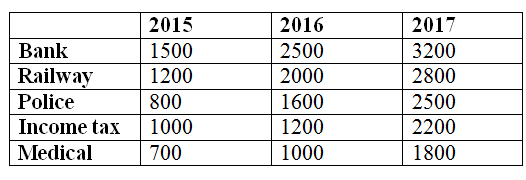# IBPS Clerk Prelims 2018 – Quantitative Aptitude Questions Day-23

Dear Readers, Bank Exam Race for the Year 2018 is already started, To enrich your preparation here we have providing new series of Practice Questions on Quantitative Aptitude – Section. Candidates those who are preparing for IBPS Clerk Prelims 2018 Exams can practice these questions daily and make your preparation effective.

[WpProQuiz 4227]

Directions (Q. 1 – 5): In each of these questions two equations (I) and (II) are given. You have to solve both the equations and give answer as,

a) If x > y

b) If x ≥ y

c) If x < y

d) If x ≤ y

e) If x = y or no relation can be established between x and y

1) I) x3 = 2744

II) y2 – 11y + 60 = 0

2) I) 3x = 5y – 4

II) 5x + 7y – 70 = 0

3) I) x2+ 12x + 32 = 0

II) 3y2 – 10y + 7 = 0

4) I) x2 = 14641

II) y = √14641

5) I) 2x2 +29x +50 =0

II) 12y2 – 7y +1 =0

Directions (Q. 6 – 10) Study the following information carefully and answer the given questions:

The following table shows the total number of government employees in 5 departments in 3 different years in a certain state.6) Total number of Bank employees in all the given years together is approximately what percentage of total number of Police in all the given years together?

a) 133 %

b) 121 %

c) 147 %

d) 159 %

e) 105 %

7) Find the difference between the total number of employees in Railway and Income tax department in the year 2015 to that of total number of employees in Bank and Medical department in the year 2017?

a) 3200

b) 2800

c) 3700

d) 2100

e) None of these

8) If the ratio of male and female employees in Income tax and medical department in the year 2017 is 6 : 5 and 4 : 5 respectively, then find the sum of male employees in Income tax and female employees in Medical department in the year 2017?

a) 2800

b) 3400

c) 3900

d) 2200

e) None of these

9) Find the average number of employees in all the given department in the year 2016?

a) 1660

b) 1780

c) 1890

d) 1520

e) None of these

10) Total number of police in the year 2016 is what percentage more than the total number of medical employees in the year 2016?

a) 50 %

b) 75 %

c) 60 %

d) 80 %

e) None of these

I) x3 = 2744

X = 14

II) y2 – 11y + 60 = 0

(y – 15) (y + 4) = 0

Y = 15, -4

Can’t be determined

3x – 5y = 4–à (1)

5x + 7y = 70–à(2)

By solving the equation (1) and (2),

X = 7, y = 5

x > y

I) x2+ 12x + 32 = 0

(x+ 4) (x+8) = 0

X = -4, -8

II) 3y2 – 10y + 7 = 0

3y2 – 3y – 7y + 7 = 0

3y(y – 1) – 7(y – 1) = 0

(3y – 7)(y – 1) = 0

Y = 7/3, 1 = 2.33, 1

x < y

I) x2 = 14641

X = +121, -121

II) y = √14641

Y = 121

x ≤ y

I) 2x2 +29x +50 = 0

2x2 +4x+25x +50 = 0

2x(x+2) + 25(x+2) = 0

(2x+25) (x+2) =0

X= -25/2, -2

II) 12y2 – 7y +1 = 0

12y2 – 4y-3y +1 = 0

4y (3y-1)-1(3y-1) = 0

(4y-1) (3y-1) = 0

Y= ¼, 1/3

X < y

Direction (6-10) :

Total number of Bank employees in all the given years together

= > 1500 + 2500 + 3200 = 7200

Total number of Police in all the given years together

= > 800 + 1600 + 2500 = 4900

Required % = (7200/4900)*100 = 147 %

The total number of employees in Railway and Income tax department in the year 2015

= > 1200 + 1000 = 2200

The total number of employees in Bank and Medical department in the year 2017

= > 3200 + 1800 = 5000

Required difference = 5000 – 2200 = 2800

The ratio of male and female employees in Income tax in 2017= 6 : 5

The ratio of male and female employees in medical department in 2017

= > 4 : 5

Total number of male employees in Income tax in the year 2017

= > 2200*(6/11) = 1200

Total number of female employees in Medical department in the year 2017

= > 1800*(5/9) = 1000

Required sum = 1200 + 1000 = 2200

The total number of employees in all the given department in the year 2016

= > 2500 + 2000 + 1600 + 1200 + 1000 = 8300

Required average = 8300/5 = 1660

Required % = [(1600 – 1000)/1000]*100 = 60 %

Kindly watch this video to know about Important DI and Application Sums

Daily Practice Test Schedule | Good Luck

 Topic Daily Publishing Time Daily News Papers & Editorials 8.00 AM Current Affairs Quiz 9.00 AM Current Affairs Quiz (Hindi) 9.30 AM IBPS Clerk Prelims – Reasoning 10.00 AM IBPS Clerk Prelims – Reasoning (Hindi) 10.30 AM IBPS Clerk Prelims – Quantitative Aptitude 11.00 AM IBPS Clerk Prelims – Quantitative Aptitude (Hindi) 11.30 AM Vocabulary (Based on The Hindu) 12.00 PM IBPS PO Prelims – English Language 1.00 PM SSC Practice Questions (Reasoning/Quantitative aptitude) 2.00 PM IBPS PO/Clerk – GK Questions 3.00 PM SSC Practice Questions (English/General Knowledge) 4.00 PM Daily Current Affairs Updates 5.00 PM IBPS PO Mains – Reasoning 6.00 PM IBPS PO Mains – Quantitative Aptitude 7.00 PM IBPS PO Mains – English Language 8.00 PM